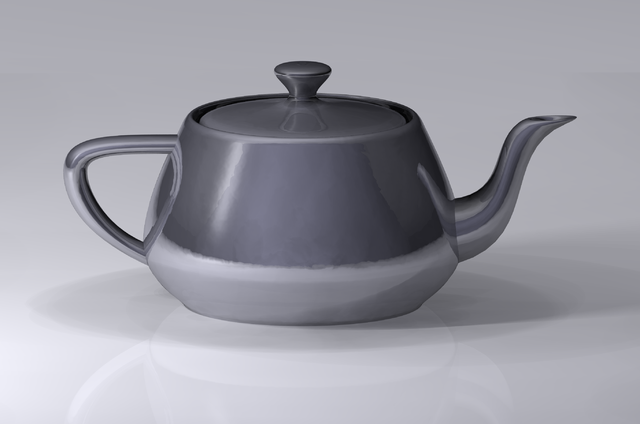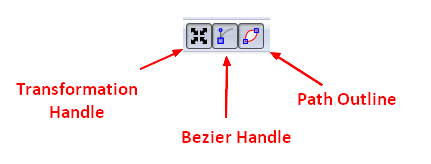# Geometry - Bezier Curve

Bézier curves are widely used in computer graphics to model smooth curves.

TrueType fonts use composite Bézier curves composed of quadratic Bézier curves.

## Structure

Bezier curves are defined by four points:

• two end points (or nodes) of the curve.
• two control points or handles,each paired with one of the end points.

The control points have the useful property that a line starting at one end of the curve and ending at the corresponding control point is tangent to the curve at the end point. This enables the smooth joining of multiple Bezier curves to form a path.

## Documentation / Reference

Discover MoreData Viz - (Timing|Easing) Function

Timing function (also known as easing) are used in transition to calculate the speed and smooth of the animation between state. They specify the rate of change of a parameter over time. Objects in real...Geometry - Shape (with transformation and notion)

in Geometry A shape is any list of line drawing commands, which strung together, describe a continuous line or closed shape that can be filled or stroked. Move, Line, Quadratic and Cubic...Inkscape - Node (Edit Path by Nodes)

Nodes define point coordinates where the path changes direction or curvature. Each segment of the selected path is subdivided into ceil (Length/Max) equal length segments. Lengths are measured in SVG...SVG - Bezier Curve

A path can be describe as a series of Bezier curves There are an infinite number of Bezier curves, but only two simple ones are available in path elements: a cubic one, called with C, and a quadratic...Statistic - Smooth (Function Continuity) (Soft ?)

To smooth a data set is to create an approximating function that attempts to capture important patterns in the data, while leaving out noise. In mathematical analysis, smoothness has to do with how many...